## Ordinary Differential Equation

An ordinary differential equation (frequently abbreviated ODE) is an equality involving a function and its Derivatives. An ODE of orderis an equation of the form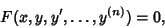(1)

where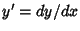is a first Derivative with respect toand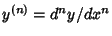is anth Derivative with respect to. An ODE of orderis said to be linear if it is of the form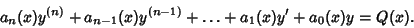(2)

A linear ODE where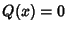is said to be homogeneous. Confusingly, an ODE of the form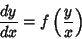(3)

is also sometimes called homogeneous.''

Simple theories exist for first-order (Integrating Factor) and second-order (Sturm-Liouville Theory) ordinary differential equations, and arbitrary ODEs with linear constant Coefficients can be solved when they are of certain factorable forms. Integral transforms such as the Laplace Transform can also be used to solve classes of linear ODEs. Morse and Feshbach (1953, pp. 667-674) give canonical forms and solutions for second-order ODEs.

While there are many general techniques for analytically solving classes of ODEs, the only practical solution technique for complicated equations is to use numerical methods (Milne 1970). The most popular of these is the Runge-Kutta Method, but many others have been developed. A vast amount of research and huge numbers of publications have been devoted to the numerical solution of differential equations, both ordinary and Partial (PDEs) as a result of their importance in fields as diverse as physics, engineering, economics, and electronics.

The solutions to an ODE satisfy Existence and Uniqueness properties. These can be formally established by Picard's Existence Theorem for certain classes of ODEs. Let a system of first-order ODE be given by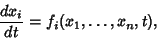(4)

for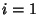, ...,and let the functions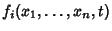, where, ...,, all be defined in a Domain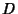of the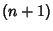-D space of the variables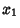, ...,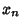,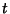. Let these functions be continuous inand have continuous first Partial Derivatives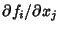for, ...,and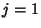, ...,in. Let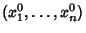be in. Then there exists a solution of (4) given by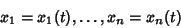(5)

for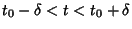(where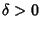) satisfying the initial conditions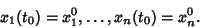(6)

Furthermore, the solution is unique, so that if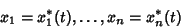(7)

is a second solution of (4) forsatisfying (6), then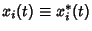for. Because everyth-order ODE can be expressed as a system offirst-order differential equations, this theorem also applies to the singleth-order ODE.

In general, anth-order ODE haslinearly independent solutions. Furthermore, any linear combination of Linearly Independent Functions solutions is also a solution.

An exact First-Order ODEs is one of the form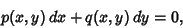(8)

where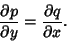(9)

An equation of the form (8) with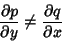(10)

is said to be nonexact. If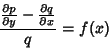(11)

in (8), it has an-dependent integrating factor. If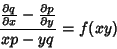(12)

in (8), it has an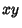-dependent integrating factor. If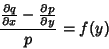(13)

in (8), it has a-dependent integrating factor.

Other special first-order types include cross multiple equations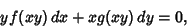(14)

homogeneous equations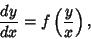(15)

linear equations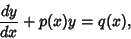(16)

and separable equations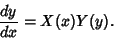(17)

Special classes of Second-Order ODEs include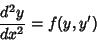(18)

(missing) and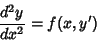(19)

(missing). A second-order linear homogeneous ODE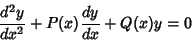(20)

for which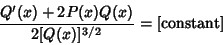(21)

can be transformed to one with constant coefficients.

The undamped equation of Simple Harmonic Motion is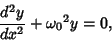(22)

which becomes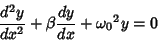(23)

when damped, and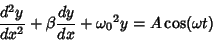(24)

when both forced and damped.

Systems with Constant Coefficients are of the form(25)

The following are examples of important ordinary differential equations which commonly arise in problems of mathematical physics.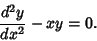(26)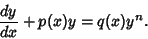(27)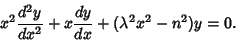(28)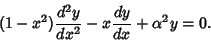(29)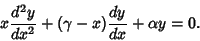(30)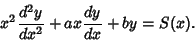(31)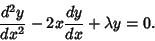(32)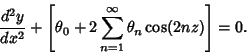(33)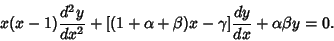(34)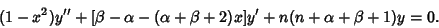(35)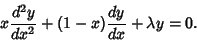(36)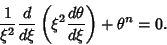(37)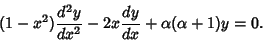(38)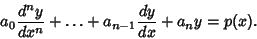(39)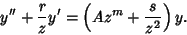(40)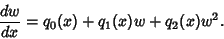(41)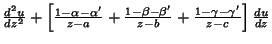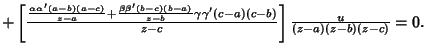(42)

See also Adams' Method, Green's Function, Isocline, Laplace Transform, Leading Order Analysis, Majorant, Ordinary Differential Equation--First-Order, Ordinary Differential Equation--Second-Order, Partial Differential Equation, Relaxation Methods, Runge-Kutta Method, Simple Harmonic Motion

ReferencesOrdinary Differential Equations

Boyce, W. E. and DiPrima, R. C. Elementary Differential Equations and Boundary Value Problems, 5th ed. New York: Wiley, 1992.

Braun, M. Differential Equations and Their Applications, 4th ed. New York: Springer-Verlag, 1993.

Forsyth, A. R. Theory of Differential Equations, 6 vols. New York: Dover, 1959.

Forsyth, A. R. A Treatise on Differential Equations. New York: Dover, 1997.

Guterman, M. M. and Nitecki, Z. H. Differential Equations: A First Course, 3rd ed. Philadelphia, PA: Saunders, 1992.

Ince, E. L. Ordinary Differential Equations. New York: Dover, 1956.

Milne, W. E. Numerical Solution of Differential Equations. New York: Dover, 1970.

Morse, P. M. and Feshbach, H. Ordinary Differential Equations.'' Ch. 5 in Methods of Theoretical Physics, Part I. New York: McGraw-Hill, pp. 492-675, 1953.

Moulton, F. R. Differential Equations. New York: Dover, 1958.

Press, W. H.; Flannery, B. P.; Teukolsky, S. A.; and Vetterling, W. T. Integration of Ordinary Differential Equations.'' Ch. 16 in Numerical Recipes in FORTRAN: The Art of Scientific Computing, 2nd ed. Cambridge, England: Cambridge University Press, pp. 701-744, 1992.

Simmons, G. F. Differential Equations, with Applications and Historical Notes, 2nd ed. New York: McGraw-Hill, 1991.

Zwillinger, D. Handbook of Differential Equations, 3rd ed. Boston, MA: Academic Press, 1997.